/

### Enterprise Risk Management Formula Book 7. Interest rates and bond pricing

7.1          Spot and forward rates

Suppose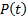is the price at time 0 of a zero-coupon bond that pays 1 at time,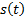is the spot rate for the period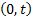, i.e. 0 to, and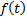is the instantaneous forward rate at time 0 for time(whereandare both continuously compounded) Then: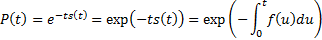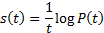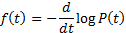If a bond gives the holder entitlements to cash flows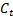at time(and is assumed not to be subject to default risk) and has a ‘dirty price’,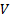, then its (gross) redemption yield (yield to maturity) is the (sensible) rate of interest that equateswith its present value, i.e.: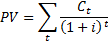Its duration is then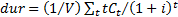and its modified duration is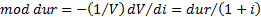.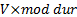is almost exactly the same as its PV01, also called DV01.

Its credit spread is the difference between its (gross) redemption yield and the corresponding yield on a reference security, often a corresponding government security providing the same cash flows in the event of non-default. The option-adjusted spread is the corresponding spread taking into account optionality in the bond in question and/or in the reference bond.

Interest rates may be expressed as annual rates,, semi-annual rates,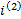, quarterly rates,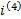, monthly rates,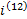or even continuously compounded rates,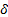, where: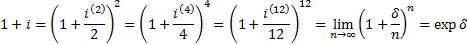The quotation convention of a bond (e.g. ACT/ACT) defines the amount of accrued interest payable when a bond is bought or sold in between coupon dates.